# Lab 5.2 ELSIF Statements

 Team-Fly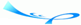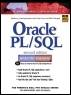Oracle® PL/SQL® Interactive Workbook, Second EditionBy Benjamin Rosenzweig, Elena Silvestrova Table of Contents Chapter 5.  Conditional Control: IF Statements

Lab Objectives

After this Lab, you will be able to:

• Use the ELSIF Statement

An ELSIF statement has the following structure:

` IF CONDITION 1 THEN     STATEMENT 1;  ELSIF CONDITION 2 THEN     STATEMENT 2;  ELSIF CONDITION 3 THEN     STATEMENT 3;  …  ELSE     STATEMENT N;  END IF; `

The reserved word IF marks the beginning of an ELSIF construct. The words CONDITION 1 through CONDITION N are a sequence of the conditions that evaluate to TRUE or FALSE. These conditions are mutually exclusive. In other words, if CONDITION 1 evaluates to TRUE, STATEMENT 1 is executed, and control is passed to the first executable statement after the reserved phrase END IF. The rest of the ELSIF construct is ignored. When CONDITION 1 evaluates to FALSE, control is passed to the ELSIF part and CONDITION 2 is evaluated, and so forth. If none of the specified conditions yield TRUE, control is passed to the ELSE part of the ELSIF construct. An ELSIF statement can contain any number of ELSIF clauses. This flow of the logic is illustrated in Figure 5.3.

##### Figure 5.3. ESLIF Statement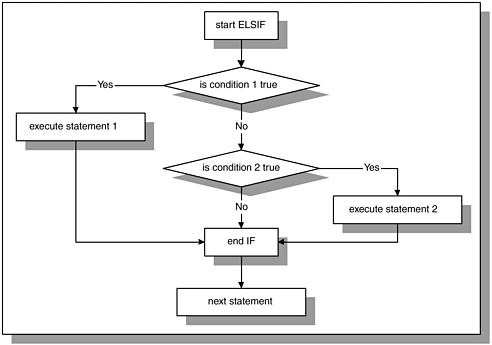Figure 5.3 shows that if condition 1 evaluates to TRUE, statement 1 is executed, and control is passed to the first statement after END IF. If condition 1 evaluates to FALSE, control is passed to condition 2. If condition 2 yields TRUE, statement 2 is executed. Otherwise, control is passed to the statement following END IF, and so forth. Consider the following example.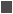FOR EXAMPLE

` DECLARE     v_num NUMBER := &sv_num;  BEGIN     IF v_num < 0 THEN        DBMS_OUTPUT.PUT_LINE (v_num||' is a negative number');     ELSIF v_num = 0 THEN        DBMS_OUTPUT.PUT_LINE (v_num||' is equal to zero');     ELSE        DBMS_OUTPUT.PUT_LINE (v_num||' is a positive number');    END IF;  END; `

The value of v_num is provided at runtime and evaluated with the help of the ELSIF statement. If the value of v_num is less that zero, the first DBMS_ OUTPUT.PUT_LINE statement executes, and the ELSIF construct terminates. If the value of v_num is greater than zero, both conditions

` v_num < 0 and v_num = 0 `

evaluate to FALSE, and the ELSE part of the ELSIF construct executes.

Assume that the value of v_num equals 5 at runtime. This example produces the following output:

` Enter value for sv_num: 5  old   2:    v_num  NUMBER := &sv_num;  new   2:    v_num  NUMBER := 5;  5 is a positive number  PL/SQL procedure successfully completed. `Remember the following information about an ELSIF statement:

• Always match IF with an END IF.

• There must be a space between END and IF. When the space is omitted, the compiler produces the following error:

` ERROR at line 22:  ORA-06550: line 22, column 4:  PLS-00103: Encountered the symbol ";" when expecting one  of the following: if `

As you can see, this error message is not very clear, and it can take you some time to correct it, especially if you have not encountered it before.

• There is no second "E" in "ELSIF".

• Conditions of an ELSIF statement must be mutually exclusive.These conditions are evaluated in sequential order, from the first to the last. Once a condition evaluates to TRUE, the remaining conditions of the ELSIF statement are not evaluated at all. Consider this example of an ELSIF construct:

` IF v_num >= 0 THEN     DBMS_OUTPUT.PUT_LINE ('v_num is greater than 0');  ELSIF v_num =< 10 THEN     DBMS_OUTPUT.PUT_LINE ('v_num is less than 10');  ELSE     DBMS_OUTPUT.PUT_LINE        ('v_num is less than ? or greater than ?');  END IF; `

Assume that the value of v_num is equal to 5. Both conditions of the ELSIF statement can evaluate to TRUE because 5 is greater than 0, and 5 is less than 10. However, once the first condition, v_num >= 0, evaluates to TRUE, the rest of the ELSIF construct is ignored.

For any value of v_num that is greater than or equal to 0 and less than or equal to 10, these conditions are not mutually exclusive. Therefore, the DBMS_OUTPUT.PUT_LINE statement associated with the ELSIF clause will not execute for any such value of v_num. In order for the second condition, v_num <= 10, to yield TRUE, the value of v_num must be less than 0.

How would you rewrite this ELSIF construct to capture any value of v_num between 0 and 10 and display it on the screen with a single condition?

When using an ELSIF construct, it is not necessary to specify what action should be taken if none of the conditions evaluate to TRUE. In other words, an ELSE clause is not required in the ELSIF construct. Consider the following example:FOR EXAMPLE

` DECLARE     v_num NUMBER := &sv_num;  BEGIN     IF v_num < 0 THEN        DBMS_OUTPUT.PUT_LINE (v_num||' is a negative number');     ELSIF v_num > 0 THEN        DBMS_OUTPUT.PUT_LINE (v_num||' is a positive number');     END IF;     DBMS_OUTPUT.PUT_LINE ('Done…');    END; `

As you can see, there is no action specified when v_num is equal to zero. If the value of v_num is equal to zero, both conditions will evaluate to FALSE, and the ELSIF statement will not execute at all. When a value of zero is specified for v_num, this example produces the following output.

` Enter value for sv_num: 0  old   2:    v_num  NUMBER := &sv_num;  new   2:    v_num  NUMBER := 0;  Done…  PL/SQL procedure successfully completed. `You probably noticed that for all IF statement examples, the reserved words IF, ELSIF, ELSE, and END IF are entered on a separate line and aligned with the word IF. In addition, all executable statements in the IF construct are indented. The format of the IF construct makes no difference to the compiler. However, the meaning of the formatted IF construct becomes obvious to us.

The IF-THEN-ELSE statement

` IF x = y THEN v_text := 'YES'; ELSE v_text := 'NO';  END  IF; `

is equivalent to

` IF x = y THEN     v_text := 'YES';  ELSE     v_text := 'NO';  END IF; `

The formatted version of the IF construct is easier to read and understand.

#### Lab 5.2 Exercises

##### 5.2.1 Use the ELSIF Statement

In this exercise, you will use an ELSIF statement to display a letter grade for a student registered for a specific section of course number 25.

Create the following PL/SQL script:

` -- ch05_3a.sql, version 1.0  SET SERVEROUTPUT ON  DECLARE     v_student_id NUMBER := 102;     v_section_id NUMBER := 89;     v_final_grade NUMBER;     v_letter_grade CHAR(1);  BEGIN     SELECT final_grade       INTO v_final_grade       FROM enrollment      WHERE student_id = v_student_id        AND section_id = v_section_id;     IF v_final_grade BETWEEN 90 AND 100 THEN        v_letter_grade := 'A';     ELSIF v_final_grade BETWEEN 80 AND 89 THEN        v_letter_grade := 'B';     ELSIF v_final_grade BETWEEN 70 AND 79 THEN        v_letter_grade := 'C';     ELSIF v_final_grade BETWEEN 60 AND 69 THEN        v_letter_grade := 'D';     ELSE        v_letter_grade := 'F';     END IF;     -- control resumes here     DBMS_OUTPUT.PUT_LINE ('Letter grade is: '||        v_letter_grade);  END; `

Note, that you may need to change the values for the variables v_student_id and v_section_id as you see fit in order to test some of your answers.

Try to answer the following questions first, and then execute the script:

 a) What letter grade will be displayed on the screen: if the value of v_final_grade is equal to 85?if the value of v_final_grade is NULL?if the value of v_final_grade is greater than 100? b) How would you change this script so that a message 'v_final_grade is null' is displayed if v_final_grade is NULL? c) How would you change this script so that student ID and section ID are provided by a user? d) How would you change the script to define a letter grade without specifying the upper limit of the final grade? In the statement, v_final_grade BETWEEN 90 and 100, number 100 is the upper limit.

#### Lab 5.2 Exercise Answers

This section gives you some suggested answers to the questions in Lab 5.2, with discussion related to how those answers resulted. The most important thing to realize is whether your answer works. You should figure out the implications of the answers here and what the effects are from any different answers you may come up with.

##### 5.2.1 Answers

 a) What letter grade will be displayed on the screen:if the value of v_final_grade is equal to 85?if the value of v_final_grade is NULL?if the value of v_final_grade is greater than 100? A1: Answer: If the value of v_final_grade is equal to 85, the value "B" of the letter grade will be displayed on the screen.The conditions of the ELSIF statement are evaluated in sequential order. The first condition ` v_final_grade BETWEEN 90 AND 100 `evaluates to FALSE, and control is passed to the first ELSIF part of the ELSIF statement. Then, the second condition ` v_final_grade BETWEEN 80 AND 89 `evaluates to TRUE, and the letter "B" is assigned to the variable v_letter_grade. Control is then passed to first executable statement after END IF, and message` Letter grade is: B `is displayed on the screen.If the value of v_final_grade is NULL, value "F" of the letter grade will be displayed of the screen.If the value of the v_final_grade is undefined or NULL, then all conditions of the ESLIF statement evaluate to NULL (notice, they do not evaluate to FALSE). As a result, the ELSE part of the ELSIF statement is executed, and letter "F" is assigned to the v_letter_grade.If the value of v_final_grade is greater than 100, value "F" of the letter grade will be displayed of the screen.The conditions specified for the ELSIF statement cannot handle a value of v_final_grade greater than 100. So, for any student whose letter grade should be A+, will result in a letter grade of "F." After the ELSIF statement has terminated, "The letter grade is: F" is displayed on the screen. b) How would you change this script so that a message 'v_final_grade is null' is displayed if v_final_grade is NULL? A2: Answer: Your script should look similar to this script. Changes are shown in bold letters.` -- ch05_3b.sql, version 2.0 SET SERVEROUTPUT ON DECLARE v_student_id NUMBER := 102; v_section_id NUMBER := 89; v_final_grade NUMBER; v_letter_grade CHAR(1); BEGIN SELECT final_grade INTO v_final_grade FROM enrollment WHERE student_id = v_student_id AND section_id = v_section_id; IF v_final_grade IS NULL THEN DBMS_OUTPUT.PUT_LINE('v_final_grade is null'); ELSIF v_final_grade BETWEEN 90 AND 100 THEN v_letter_grade := 'A'; ELSIF v_final_grade BETWEEN 80 AND 89 THEN v_letter_grade := 'B'; ELSIF v_final_grade BETWEEN 70 AND 79 THEN v_letter_grade := 'C'; ELSIF v_final_grade BETWEEN 60 AND 69 THEN v_letter_grade := 'D'; ELSE v_letter_grade := 'F'; END IF; -- control resumes here DBMS_OUTPUT.PUT_LINE ('Letter grade is: '|| v_letter_grade); END; `One more condition has been added to the ELSIF statement. The condition` v_final_grade BETWEEN 90 AND 100 `becomes the first ELSIF condition. Now, if the value of v_final_grade is NULL, the message "v_final_grade is null" is displayed on the screen. However, there is no value assigned to the variable v_letter_grade. The message "Letter grade is:" is displayed on the screen as well. c) How would you change this script so that student ID and section ID are provided by a user? A3: Answer: Your script should look similar to this script. Changes are shown in bold letters.` -- ch05_3c.sql, version 3.0 SET SERVEROUTPUT ON DECLARE v_student_id NUMBER := &sv_student_id; v_section_id NUMBER := &sv_section_id; v_final_grade NUMBER; v_letter_grade CHAR(1); BEGIN SELECT final_grade INTO v_final_grade FROM enrollment WHERE student_id = v_student_id AND section_id = v_section_id; IF v_final_grade BETWEEN 90 AND 100 THEN v_letter_grade := 'A'; ELSIF v_final_grade BETWEEN 80 AND 89 THEN v_letter_grade := 'B'; ELSIF v_final_grade BETWEEN 70 AND 79 THEN v_letter_grade := 'C'; ELSIF v_final_grade BETWEEN 60 AND 69 THEN v_letter_grade := 'D'; ELSE v_letter_grade := 'F'; END IF; -- control resumes here DBMS_OUTPUT.PUT_LINE ('Letter grade is: '|| v_letter_grade); END; ` d) How would you change the script to define a letter grade without specifying the upper limit of the final grade? In the statement, v_final_grade BETWEEN 90 and 100, number 100 is the upper limit. A4: Answer: Your script should look similar to following. Changes are shown in bold letters.` -- ch05_3d.sql, version 4.0 SET SERVEROUTPUT ON DECLARE v_student_id NUMBER := 102; v_section_id NUMBER := 89; v_final_grade NUMBER; v_letter_grade CHAR(1); BEGIN SELECT final_grade INTO v_final_grade FROM enrollment WHERE student_id = v_student_id AND section_id = v_section_id; IF v_final_grade >= 90 THEN v_letter_grade := 'A'; ELSIF v_final_grade >= 80 THEN v_letter_grade := 'B'; ELSIF v_final_grade >= 70 THEN v_letter_grade := 'C'; ELSIF v_final_grade >= 60 THEN v_letter_grade := 'D'; ELSE v_letter_grade := 'F'; END IF; --- control resumes here DBMS_OUTPUT.PUT_LINE ('Letter grade is: '|| v_letter_grade); END; `In this example, there is no upper limit specified for the variable v_final_grade because the BETWEEN operator has been replaced with ">=" operator. Thus, this script is able to handle a value of v_final_grade that is greater than 100. Instead of assigning letter "F" to v_letter_grade (in version 1.0 of the script), the letter "A" is assigned to the variable v_letter_grade. As a result, this script produces more accurate results.

#### Lab 5.2 Self-Review Questions

In order to test your progress, you should be able to answer the following questions.

Answers appear in Appendix A, Section 5.2.

 1) An ELSIF construct can have only one ELSIF clause present. _____ True_____ False 2) There are multiple ELSE clauses present in an ELSIF construct. _____ True_____ False 3) What part of the ELSIF statement is executed when all of the conditions specified evaluate to NULL? _____ IF part_____ One of the ELSIF parts_____ ELSE part_____ ELSIF statement is not executed at all 4) When the conditions of the ELSIF statement are not mutually exclusive, which of the following occur? _____ ELSIF statement causes an error._____ ELSIF statement is not executed at all._____ Statements associated with the first condition that evaluates to TRUE are executed._____ Statements associated with the last condition that evaluates to TRUE are executed. 5) An ELSIF statement without the ELSE part causes a syntax error. _____ True_____ False

 Team-FlyTop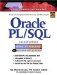Oracle PL/SQL Interactive Workbook (2nd Edition)
ISBN: 0130473200
EAN: 2147483647
Year: 2002
Pages: 146

Similar book on Amazon

flylib.com © 2008-2017.
If you may any questions please contact us: flylib@qtcs.net